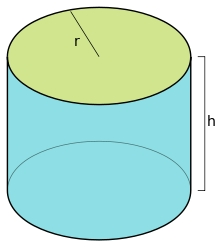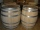# Bottle

A company wants to produce a bottle whose capacity is 1.25 liters. Find the dimensions of a cylinder that will be required to produce this 1.25litres if the hight of the cylinder must be 5 times the radius.

Correct result:

r =  0.4301 dm
h =  2.1506 dm

#### Solution:We would be pleased if you find an error in the word problem, spelling mistakes, or inaccuracies and send it to us. Thank you!Tips to related online calculators
Do you know the volume and unit volume, and want to convert volume units?

## Next similar math problems:

• Cylinder twiceIf the radius of the cylinder increases twice, and the height is reduced twice, then the volume of the cylinder increases (how many times?):
• Thousand ballsWe have to create a thousand balls from a sphere with a diameter of 1 m. What will be their radius?
• Perimeter of baseThe circumference of the base of the rotating cylinder is same as its height. What is the diameter and height of this cylinder with volume 1 liter?
• Two cylindersTwo cylinders are there one with oil and one with an empty oil cylinder has no fixed value assume infinitely. We are pumping out the oil into an empty cylinder having radius =1 cm height=3 cm rate of pumping oil is 9 cubic centimeters per sec and we are p
• Two rectangular boxesTwo rectangular boxes with dimensions of 5 cm, 8 cm, 10 cm, and 5 cm, 12 cm, 1 dm are to be replaced by a single cube box of the same cubic volume. Calculate its surface.
• ContainerThe container has a cylindrical shape the base diameter 0.8 m and the area of the base is equal to the area of the wall. How many liters of water can we pour into the container?
• A residentialA residential colony has a population of 5400 and 60 litres of water is required per person per day. For the effective utilization of rain water, they constructed a water reservoir measuring 48m × 27m × 25m to collect the rain water. For how many days, th
• Cylinder containerThe cylindrical container with a diameter of 1.8 m contains 2,000 liters of water. How high does the water reach?
• Water flow 2How many litres of water will flow in 7 minutes from a cylindrical pipe 1 cm in diameter, if the water flows at a speed of 30 km per hour
• An oilAn oil drum is cut in half. One half is used as a water trough. Use the dimensions; length 82cm, width 56cm to estimate the capacity of the water trough in liters.
• Water tankWhat is the height of the cuboid-shaped tank with the bottom dimensions of 80 cm and 50 cm if the 480 liters of water reaches 10 cm below the top?
• The potThe pot is a cylinder with a volume of V = 7l and an inner diameter of d = 20cm. Find its depth.
• Water in aquariumThe aquarium cuboid shape with a length of 25 cm and a width of 30 cm is 9 liters of water. Calculate the areas which are wetted with water.
• Fuel economyHow many kilometers is sufficient petrol in the cylinder fuel tank with a diameter 40 cm and the base of tank length 1 m, when it is filled to 60% and if the car consume 15 liters per 100 km?
• Cylindrical tankCylindrical tank holds 600hl water and is deep 2.5 m. Calculate the diameter of the cylinder.
• A cylindrical tankA cylindrical tank can hold 44 cubic meters of water. If the radius of the tank is 3.5 meters, how high is the tank?
• Cuboid aquariumCuboid 25 times 30 cm. How long is third side if cuboid contains 30 liters of water?